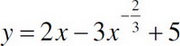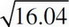# AP Calculus AB Practice Test 24

### Test Information8 questions24 minutes

Calculator Allowed

1. An open top cylinder has a volume of 125π in3. Find the radius required to minimize the amount of material to make the cylinder.

2. If the position of a particle is given by x(t) = 2t3 - 5t2 + 4t + 6, where t > 0. What is the distance traveled by the particle from t = 0 to t = 3 ?

3. At what times, t, are the x- and y-components of the particle's velocity equal if the curve is represented by x = 2t3 + 3t2 - 5 and y = t4 - 4t3 + 7t2?

4. Find the equation of the line tangent to the graph ofat x = 8.

5. Which point on the curve y = 5x3 - 12x2 - 12x + 64 has a tangent that is parallel to y = 3 ?

6. A 50 foot ladder is leaning against a building and being pulled to the ground, so the top is sliding down the building. If the rate the bottom of the ladder is being pulled across the ground is 12 ft/sec, what is the rate of the top of the ladder sliding down the building when the top is 30 ft from the ground?

7. The tangent to a curve described by x = 3t3 - 5t + 2 and y = 7t2 - 16 is what at t = 1?

8. Approximate.